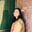Related Tags

c

# What is cpowf() in C?Umme Ammara

The cpowf function is a C library function that raises a complex number to a complex power.

The following illustration shows the functionality of the cpowf function:

To use the cpowf function, we need to include the complex.h header file, as shown below:

#include <complex.h>


## Syntax

The cpowf function takes two arguments and returns the complex power. It is declared as follows:

## Parameters

The cpowf function takes two arguments of the float complex type.

• The first parameter is the number we want to raise

• The second parameter is the power with which we want to raise the number

## Return value

The cpowf function computes and returns the power in the float complex type.

## Example

The code below shows the use of cpowf function in C:

#include <stdio.h>
#include <complex.h>

int main() {

//Declare x and y
float complex x  = 1.0+2.0*I;
float complex y = 3.0;

//Call cpowf i.e x^y
float complex ans = cpowf(x,y);

//Display result
printf ("(1+2i)^3 = %f%+fi\n", creal(ans), cimag(ans) );

return 0;
}

RELATED TAGS

c

CONTRIBUTORUmme Ammara